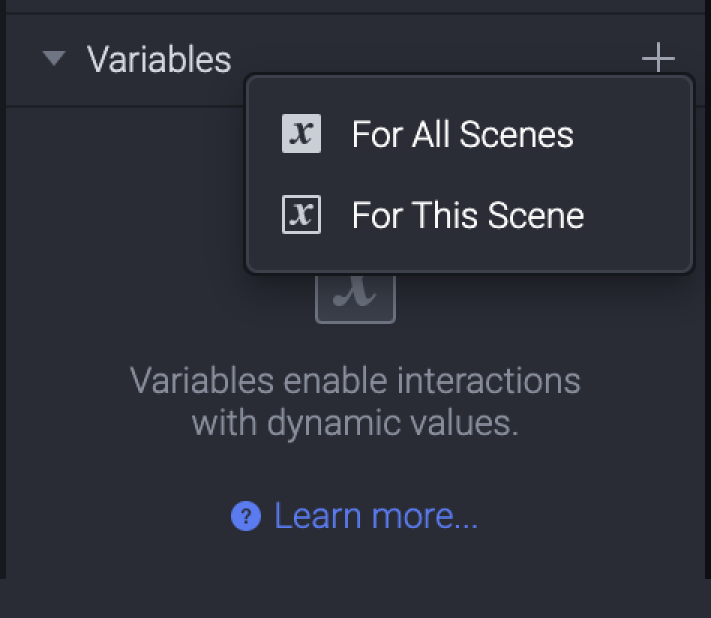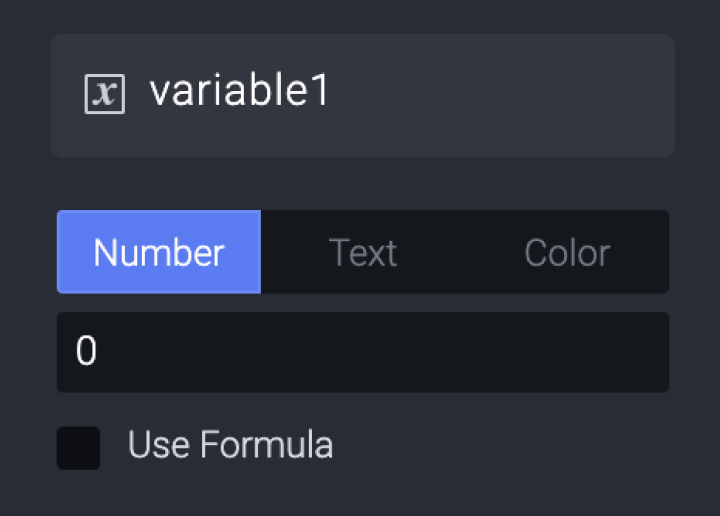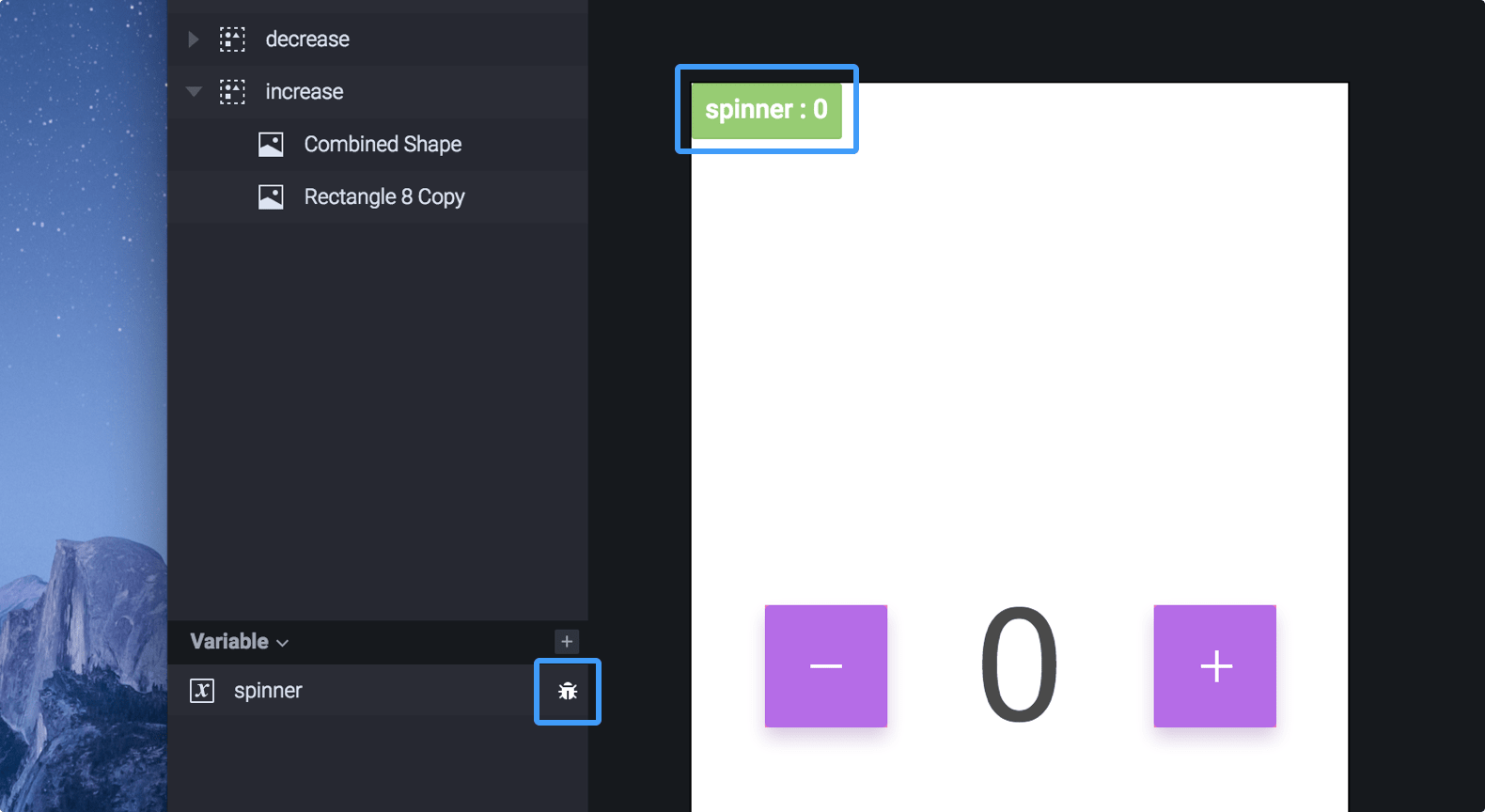# Variables

Think of variables as "baskets" that store values. Store any data that you might want to use later, e.g., a name, password, or account balance. Modify the value in a variable and detect any changes to a variable for dynamic interactions. Use variables anywhere you can use formulas.

Completely new to variables? Jumpstart with the examples below and the other sections to get the most out of variables.

• Predefined variables — A set of variables that are accessible anywhere. They automatically change dynamically as they always store the latest value.
• Use cases — Practical use cases on variables to learn from.

## Difference Between Variables & Formulas

First and foremost, formulas and variables do not exclude each other as you can use them independently from another. But, they do complement each other.

Think of a variable as a "basket" that stores a value. As a formula is an expression that "returns" a result, you might want to store this result to be used later. That's where variables come into play. To use the value stored in a variable, you need formulas.

To take it a step further, a variable could store an entire formula (instead of just the result). You would do this if you want to make a formula reusable across a scene.

Long story short, a formula could contain variables while a variable could contain a formula as well.

## Choosing the Variable Scope

When creating a variable, there are two variable scopes to choose from. The scope indicates where you can use the variable.

1.### For All Scenes

A variable for all scenes is usable in all scenes in a prototype. Use this for "passing" data between scenes. If the value of a variable for all scenes changes in Scene A, the changes in the variable would be visible in Scene B as well.

### For This Scene

A variable for this scene is usable only in the scene where you created the variable. This means that you cannot use a variable in Scene B when you created it in Scene A.

It's possible to enter a formula when creating a variable for this scene. This way, make formulas easily reusable across a scene.

## Using Data Types

A variable can have one of the following three data types: text, number, or color.

1.### Text

A text variable stores text as a value. If you use a number as a value, the text variable will treat it as a text.

### Number

A number variable stores numbers as a value. Anything that's not a number won't work.

### Color

A color variable stores a hex color code as a value. Anything that's not a hex color code won't work.

## Using Assign & Detect

There is one trigger and one response that you often use with variables.

### Assign Response

Assign a value to a variable. A value could be of the text, number, or color data type. Moreover, a value could be fixed or the result of a formula.

### Detect Trigger

Continuously detect any changes to a variable. Trigger any response based on whatever change took place to a variable.

## Displaying Variables

To see the value of a variable, display variables in the scene on the canvas and in the preview window. To enable this, click on the bug icon next to the variable name in the variable panel.

For debugging purposes, this is the way to see if a variable is storing the correct value.## Learning the Basics

Variables don't have to be overwhelming. Once you have created your first interaction with variables, you'll be on your way to make more dynamic interactions.

In this video, learn how to make an interaction involving a rectangle layer moving based on the value of a variable changing.

## Practicing with Examples

### Making a Spinner

To make a simple spinner, try using variables. This example shows you how to increase or decrease the value of a variable by 1. The text layer will then display the value of the variable—every time it changes.### Making a Ripple Effect

To make a ripple effect, use some of the available predefined variables. This example shows you how to make the ripple effect at the exact position where a touch takes place.